diff_months: 11

# IFY Mathematics Assignment

Flat 50% Off Order New Solution
Order Code:
• Country :

United Kingdom

Section A

Answer ALL questions. This section carries 40 marks.

Question A1
Line l passes through the points (?p, 3) and (2, 3p).
Line l is perpendicular to the line with equation 2x + 3y + 4 = 0.
Find the value of p.

Question A2
A box holds 6 milk chocolates and 10 plain chocolates. Two chocolates are
drawn from the box one after the other with no replacement.
Find the probability that both chocolates are milk or both chocolates are plain.

Question A3
(a) Factorise 21x^2 + 5x ? 6.

(b) Use your answer to part (a) to solve the equation 21x^2 + 5x ? 6.

Give your answers in the form a/b where a and b are integers.

Question A4
In the expansion of (3 + kx)^5,, find the value of k if the coefficient of the x^2 term is 1.5 times larger than the coefficient of the x^3 term.

Question A5
On the same axes, sketch the graphs of y = e^x and y = e^?x (this must not be done on graph paper).
On your sketch, show clearly the coordinates of any point where your graphs cross the x ? axis or y ? axis.

Question A6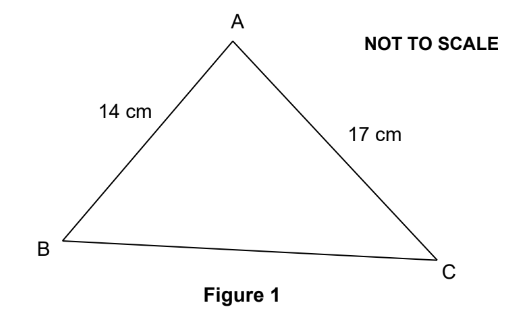Figure 1 shows the acute-angled triangle ABC with AB = 14 cm and AC = 17 cm.
The area of triangle ABC is 114 cm^2
figures.
In this question, 1 mark will be given for the correct use of significant figures.

Question A7
A curve has equation y = x^3 ? 12x^2 + 48x ? 64.

Find the coordinates of the stationary value and show that it is a point of inflexion.

Question A8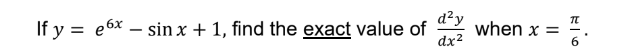All working must be shown.

Question A9
Find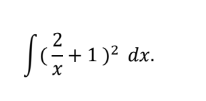Question A10
Find the value of p if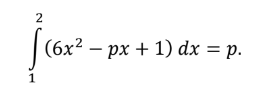Section B

This section carries 60 marks.

Question B1
(a) You are given (x ? 9) is a factor of f(x) = x^3 + qx^2 ? 27x.

i. Use the Factor Theorem to find the value of q.

ii. Divide f(x) by (x ? 9).

iii. Hence factorise f(x) completely.

(b) Use substitution to solve the simultaneous equations x + 2y = ?9
2x^2 ? y^2 = 1

(c) The line with equation 3x ? 4y = 12 crosses the x ? axis at point X and the y ? axis at point Y.
Find the equation of the line which passes through the mid-point of XY and is perpendicular to XY.
Give your answer in the form ax + by + c = 0 where a, b and c are integers.]

Question B2
(a) On her way to college, a student passes two sets of traffic lights. The
probability that she is stopped at both sets of traffic lights is 0.6.
i. Find the probability that she is stopped at the second set if the
probability that she is stopped at the first set is 0.8.

ii. Find the probability that, on 3 consecutive days, she is stopped at both
sets of traffic lights on all 3 days.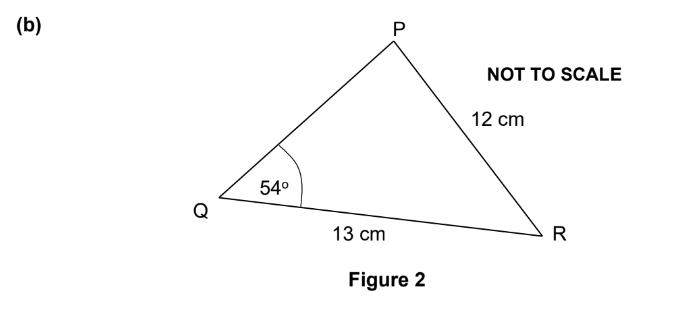Figure 2 shows the acute-angled triangle PQR with PR = 12 cm,
QR = 13 cm and angle Q = 54o
.

Find angle P in degrees. [ 2 ]

ii. Use the cosine rule to find the length of PQ. [ 4 ]

(c) Solve the equation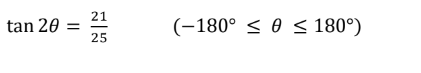Question B3
(a) An arithmetic series has common difference 2.5 and the 25th term is 6 times larger than the first term.
i. Find the first term. [ 2 ]
ii. Find the sum of the first 80 terms. [ 3 ]

(b) The 4th term of a geometric series is 27000 and the 7th term is 15625.
i. Find the common ratio and the first term. The first term must be given
in full with no rounding off.

ii. Find which term in the series is the first to fall below 800. [ 3 ]

iii. Show that the sum of the series will never reach 280000. [ 2 ]

Question B4
(a)Two variables, N and t, are connected by the formula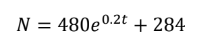i.Two variables, N and t, are connected by the formula  N = 480e^0.2t + 284 State the value of N when t = 0.

ii. Find the value of t when N = 1000.
iii. Find the value of dN/dt when t = 3. Working must be shown.

iv. What happens to N as t becomes large?

(b) Solve the equation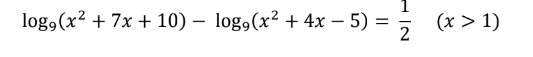All working must be shown.

(c) Find the value of k if

Question B5
(a)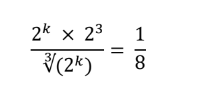(b)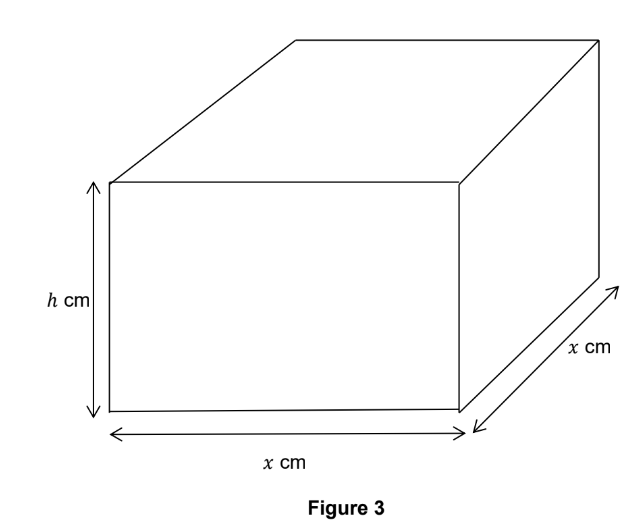Figure 3 shows a solid cuboid with a square base of side x cm and
height h cm. The sum of the lengths of all the edges is 840 cm.

i. Write h in terms of x.

ii. Show that the surface area, A, of the cuboid is given by

A = 840x ? 6x^2
. iii. Use calculus to find the value of x which gives the maximum surface
area.

iv. Confirm that your value of x gives a maximum.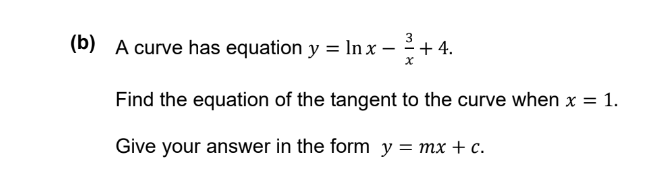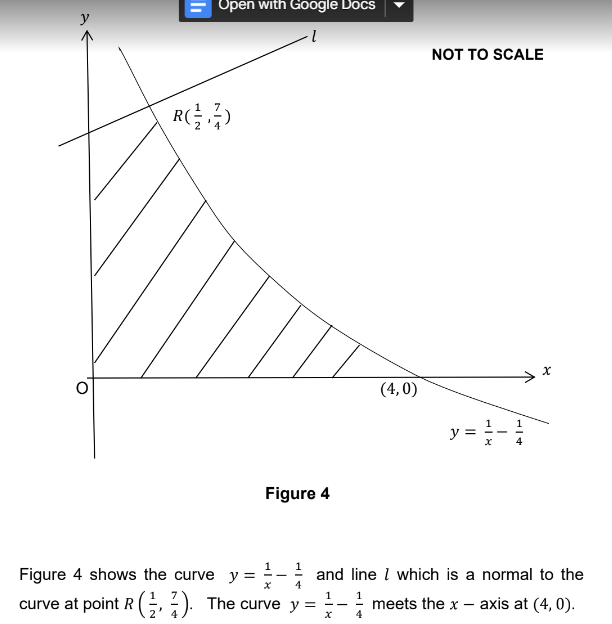(a)

Find the equation of line l giving your answer in the form y = mx + c. [ 4 ]

(b) Find the area, which is shaded on the diagram, that is bounded by line l, the
curve y and both axes.
where a and b are integers.

(c) Point S lies on the curve y  such that the tangent to the curve at point S is parallel to the line with equation 16x + 25y + 1 = 0.
Find the two possible sets of coordinates of point S.

• Uploaded By : Katthy Wills
• Posted on : January 12th, 2023
• Views : 217

## Order New Solution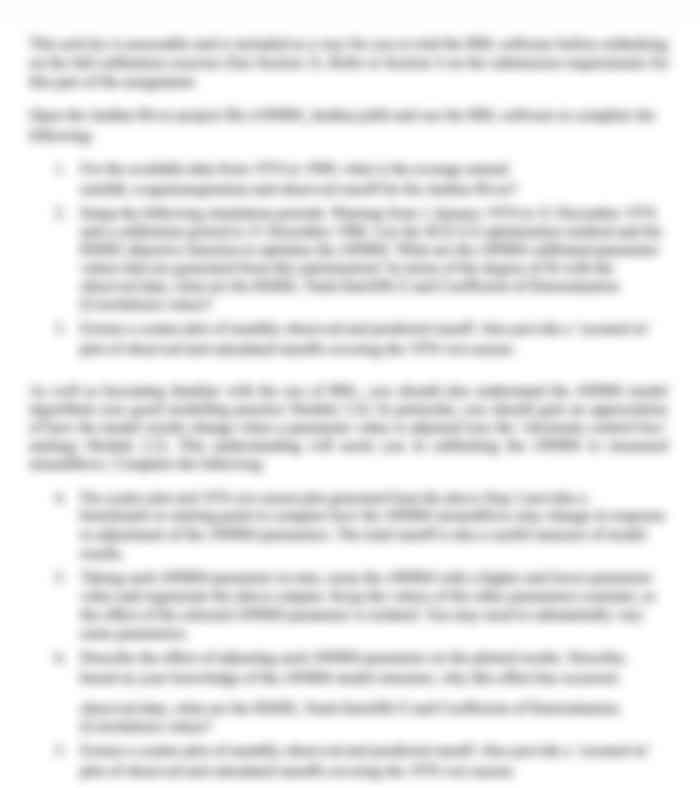## Choose a Plan

80 USD
• All in Gold, plus:
• 30-minute live one-to-one session with an expert
• Understanding Marking Rubric
• Structuring & Formatting
• Referencing & Citing
Most
Popular

### Gold

30 50 USD
• Get the Full Used Solution
(Solution is already submitted and 100% plagiarised.
Can only be used for reference purposes)
Save 33%

### Silver

20 USD
• Journals
• Peer-Reviewed Articles
• Books
• Various other Data Sources – ProQuest, Informit, Scopus, Academic Search Complete, EBSCO, Exerpta Medica Database, and more